Examples

Chapter 9 Class 11 Straight Lines
Serial order wise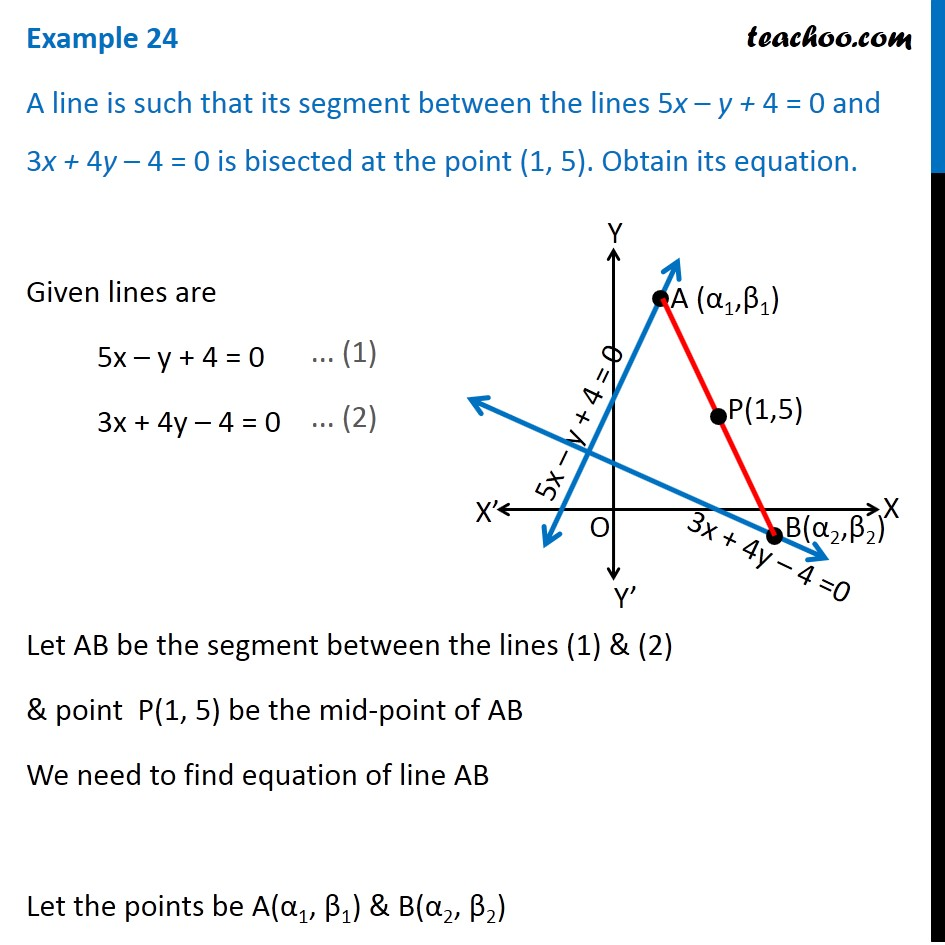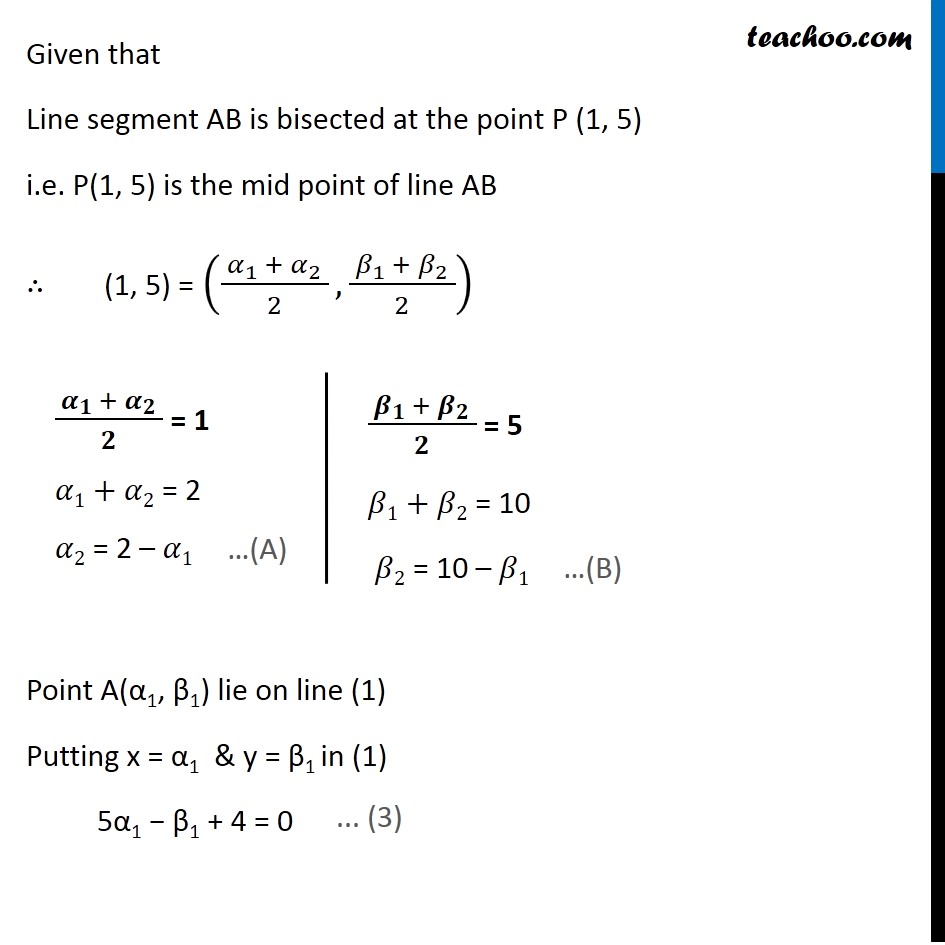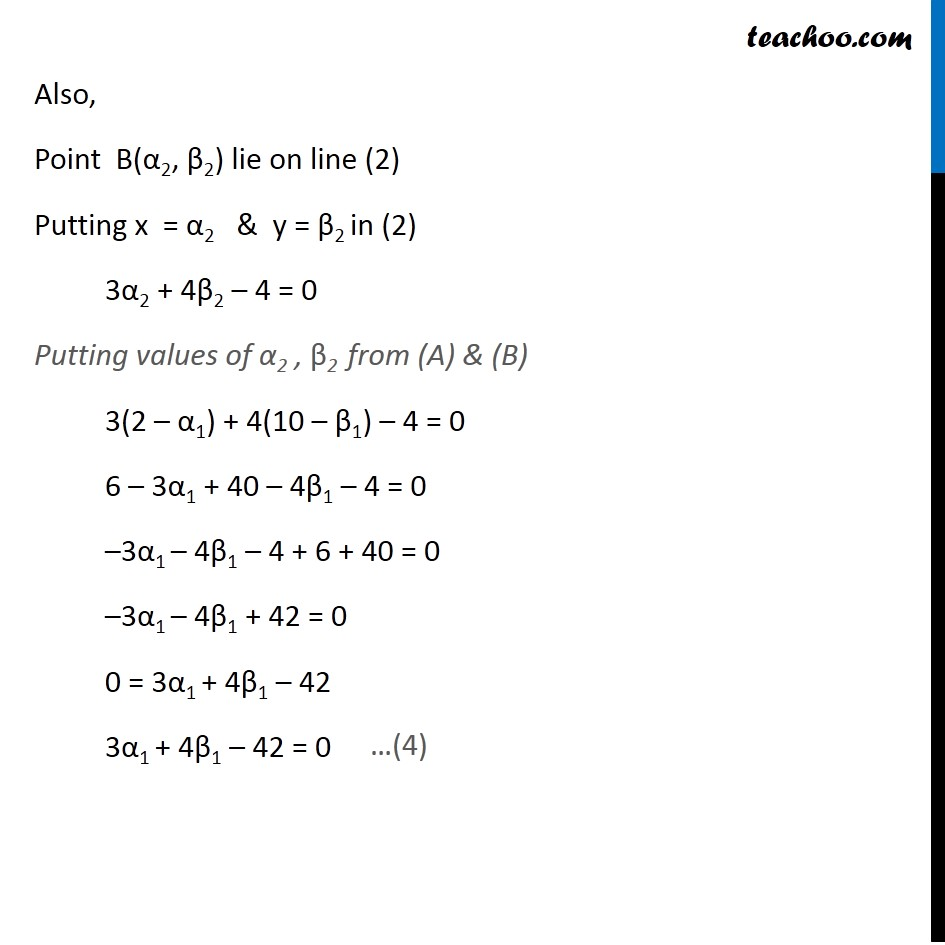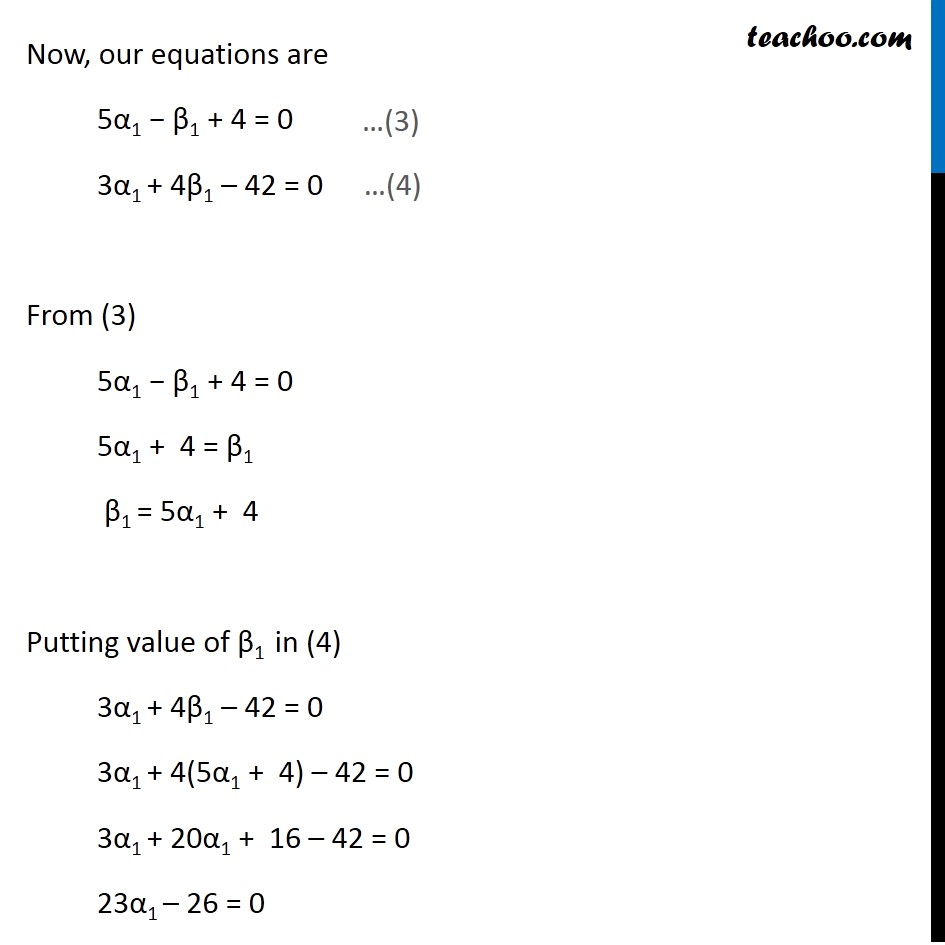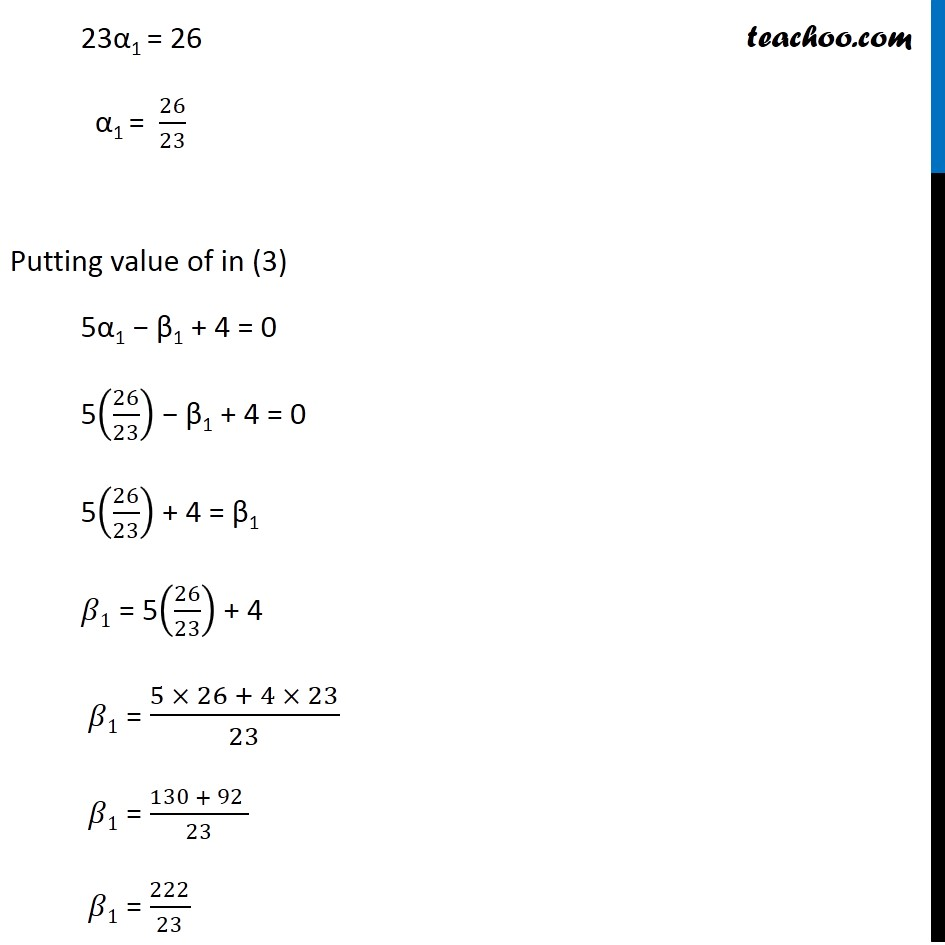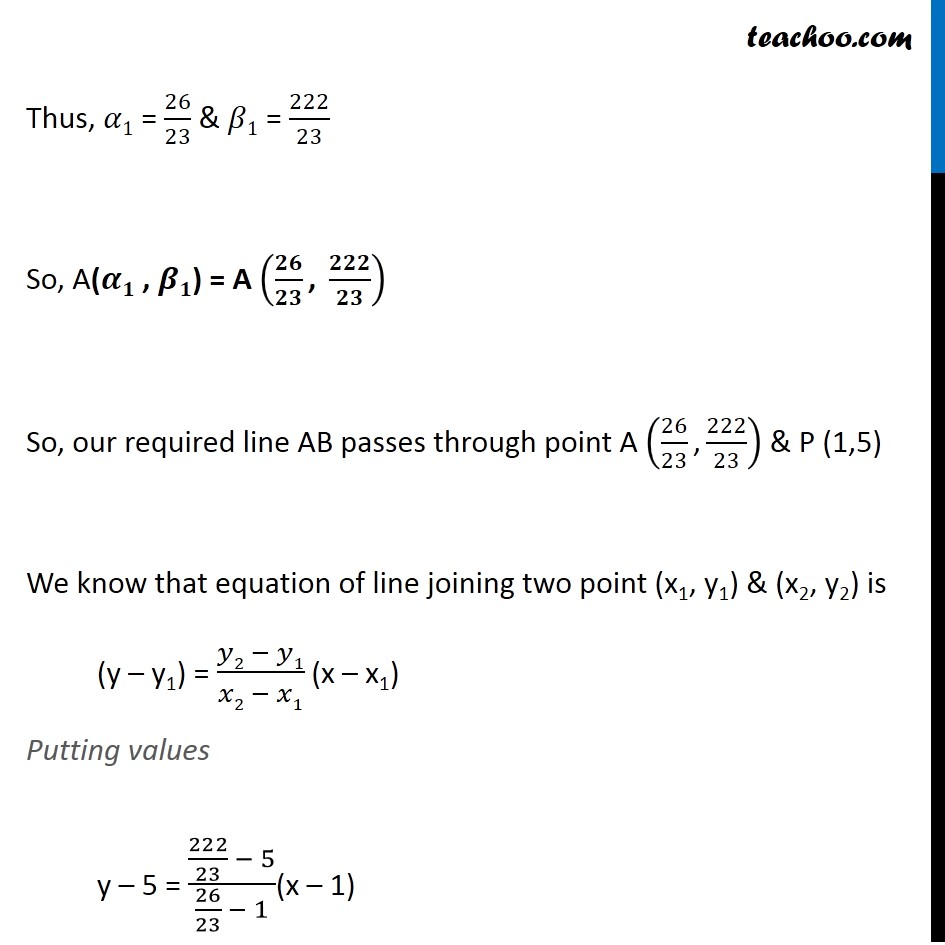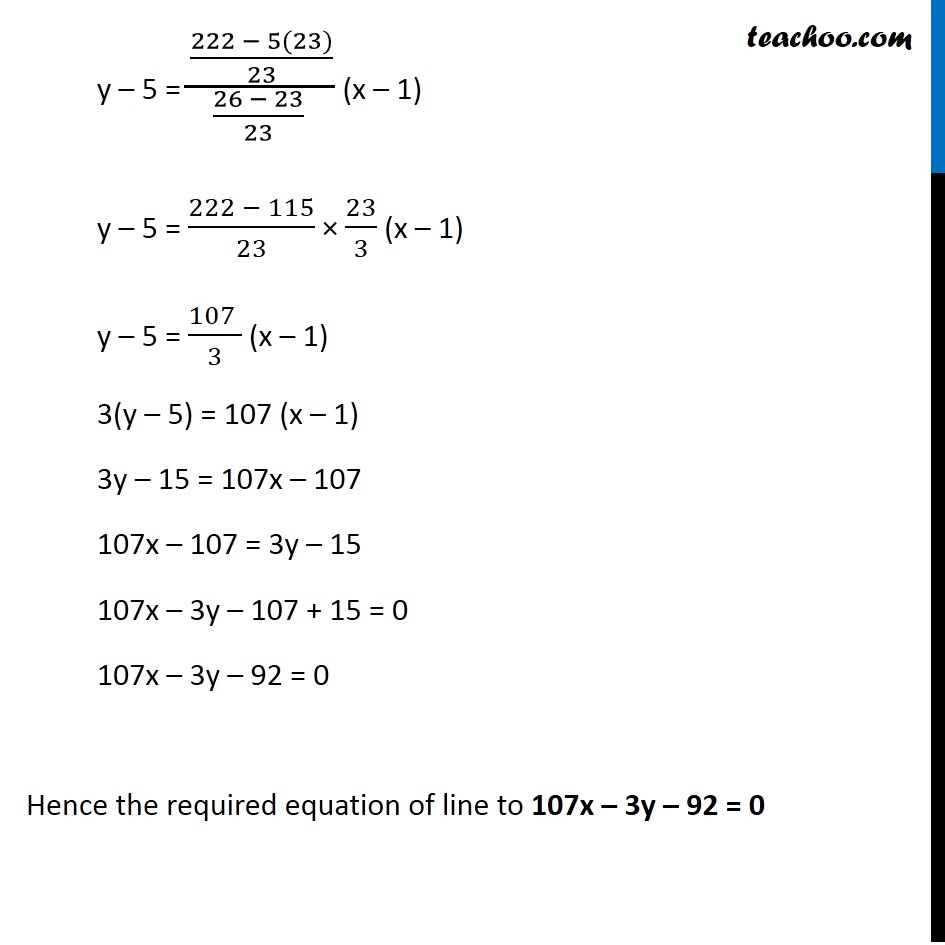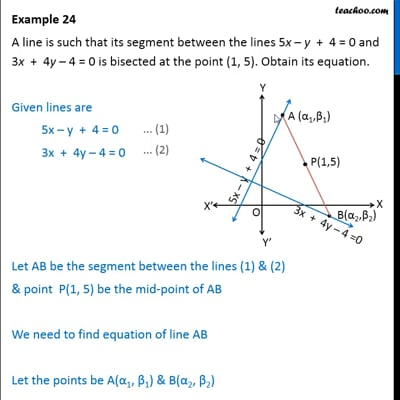This video is only available for Teachoo black users

Learn in your speed, with individual attention - Teachoo Maths 1-on-1 Class

### Transcript

Example 15 A line is such that its segment between the lines 5x – y + 4 = 0 and 3x + 4y – 4 = 0 is bisected at the point (1, 5). Obtain its equation. Given lines are 5x – y + 4 = 0 3x + 4y – 4 = 0 Let AB be the segment between the lines (1) & (2) & point P(1, 5) be the mid-point of AB We need to find equation of line AB Let the points be A(α1, β1) & B(α2, β2) Given that Line segment AB is bisected at the point P (1, 5) i.e. P(1, 5) is the mid point of line AB ∴ (1, 5) = (( 𝛼_(1 )+ 𝛼_2 )/2,( 𝛽_(1 )+ 𝛽_2 )/2) a Point A(α1, β1) lie on line (1) Putting x = α1 & y = β1 in (1) 5α1 − β1 + 4 = 0 ( 𝜶_(𝟏 )+ 𝜶_𝟐 )/𝟐 = 1 𝛼1+𝛼2 = 2 𝛼2 = 2 – 𝛼1 ( 𝜷_(𝟏 )+ 𝜷_𝟐 )/𝟐 = 5 𝛽1+𝛽2 = 10 𝛽2 = 10 – 𝛽1 Also, Point B(α2, β2) lie on line (2) Putting x = α2 & y = β2 in (2) 3α2 + 4β2 – 4 = 0 Putting values of α2 , β2 from (A) & (B) 3(2 – α1) + 4(10 – β1) – 4 = 0 6 – 3α1 + 40 – 4β1 – 4 = 0 –3α1 – 4β1 – 4 + 6 + 40 = 0 –3α1 – 4β1 + 42 = 0 0 = 3α1 + 4β1 – 42 3α1 + 4β1 – 42 = 0 Now, our equations are 5α1 − β1 + 4 = 0 3α1 + 4β1 – 42 = 0 From (3) 5α1 − β1 + 4 = 0 5α1 + 4 = β1 β1 = 5α1 + 4 Putting value of β1 in (4) 3α1 + 4β1 – 42 = 0 3α1 + 4(5α1 + 4) – 42 = 0 3α1 + 20α1 + 16 – 42 = 0 23α1 – 26 = 0 23α1 = 26 α1 = 26/23 Putting value of in (3) 5α1 − β1 + 4 = 0 5(26/23) − β1 + 4 = 0 5(26/23) + 4 = β1 𝛽1 = 5(26/23) + 4 𝛽1 = (5 × 26 + 4 × 23)/23 𝛽1 = (130 + 92 )/23 𝛽1 = 222/23 Thus, 𝛼1 = 26/23 & 𝛽1 = 222/23 So, A(𝜶𝟏 , 𝜷𝟏) = A (𝟐𝟔/𝟐𝟑 ", " 𝟐𝟐𝟐/𝟐𝟑) So, our required line AB passes through point A (26/23 "," 222/23) & P (1,5) We know that equation of line joining two point (x1, y1) & (x2, y2) is (y – y1) = (𝑦2 − 𝑦1)/(𝑥2 − 𝑥1) (x – x1) Putting values y – 5 = (222/23 − 5)/(26/23 − 1)(x – 1) y – 5 = ((222 − 5(23))/23)/((26 − 23)/23 ) (x – 1) y – 5 = (222 − 115)/23 × 23/3 (x – 1) y – 5 = (107 )/3 (x – 1) 3(y – 5) = 107 (x – 1) 3y – 15 = 107x – 107 107x – 107 = 3y – 15 107x – 3y – 107 + 15 = 0 107x – 3y – 92 = 0 Hence the required equation of line to 107x – 3y – 92 = 0